If you have a related question, please click the "Ask a related question" button in the top right corner. The newly created question will be automatically linked to this question.

# [FAQ] Isolated Gate Drivers: Why Does OUTH Have A Higher Effective Resistance Compared To OUTL?

Other Parts Discussed in Thread: UCC53X0S

How does the hybrid pull-up structure impact the gate driver?

• One care about for gate drivers how much power get dissipated inside of the chip to maintain temperature range. This is a factor of the internal chip, the output stage in some of our gate drivers features a hybrid pullup structure that allows efficient current delivery during the Miller plateau to achieve low switching losses for the power MOSFET or IGBT. The hybrid pullup output, OUTH, consists of a P-channel MOSFET connected in parallel with a N-channel MOSFET. To understand the effective resistance of the hybrid pullup structure, we need to understand the basic operating mode conditions of a MOSFET.

• Operational modes of a MOSFET: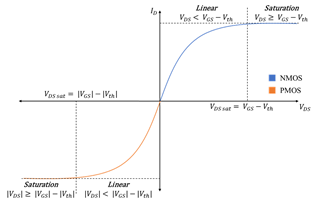Figure 1: NMOS vs PMOS Operation Mode

Figure 1 shows the I-V curves of the N-channel, NMOS, and P-channel, PMOS, MOSFETs. Using NMOS as an example, there are three regions that can be shown from the I-V curve. When the voltage from gate to source, VGS, is below the threshold voltage, VTH, the device will be in the cutoff mode, (VGS < VTH). In this region the device will be off and won’t conduct any current.

When the voltage from drain to source, VDS, is less than the difference between VGS and VTH the NMOS will be in the Linear Region, (VDS < VGS - VTH).

When the VDS is greater than the difference between VGS and VTH it will be in the saturation mode, (VDS > VGS - VTH), where the MOSFET is acting like a current source and dissipates the most power.

The main difference between the NMOS and the PMOS is the gate voltage. The PMOS is driven with a negative voltage while the NMOS is driven with a positive voltage, but this is invisible outside of the gate driver. The simplest version of an output stage for a gate driver is using a PMOS as the turn on device, the pullup, and the NMOS as the turn off device, pulldown. The PMOS is optimal for the pullup because when it is fully turned-on, there is no diode-drop when the output is clamped to the supply rail. With an NMOS by itself, there could be a drop depending on how the gate is controlled, such as with a charge pump circuit. However, based on the PMOS characteristics it is typically larger in size compared to the NMOS for the same current rating. Thus, a hybrid pullup structure is used in many of TI’s gate driver chips to provide high output current with smaller size.

• Hybrid Pull-up Structure:Figure 2: Output Stage --- UCC53x0S

The figure above shows the output stage of a split output gate driver, which means the turn-on pin is separated from the turn-off pin. As mentioned before, gate drivers that use the hybrid pull-up structure utilize the paralleled NMOS and PMOS to provide high peak current for a fast turn-on. With the pull-down, an NMOS by itself can be used because it is easily controlled since the gate is referenced to VEE2.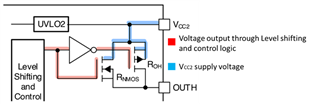Figure 3: Hybrid Pull-up structure--- UCC53x0S

Let’s look at how the hybrid pullup works during turn-on, shown in Figure 3.  Initially, OUTH is low. When the voltage passes the level shifting and control logic, it will take two paths. The first one is through the inverting gate, representing the driver of the PMOS gate. With VCC2 applied, the PMOS will function in the linear operation mode, . The second path is through the NMOS, and with the VCC2 applied and OUTH initially low, the voltage through the gate source is going to be higher than the threshold voltage which will lead to the NMOS to operate in the linear operation,, as well. Thus, the turn-on phase output resistance is the parallel combination ROH || RNMOS.

Figure 4 shows RDS(ON) vs VGS. The on-resistance of the NMOS increases as VGS becomes smaller, i.e. as the MOSFET moves into the cutoff region as OUTH rises. On the other hand, the PMOS on- resistance is maintained as OUTH rises since its gate voltage is referenced to VCC2. This helps deliver the highest peak-source current when it is most needed during the Miller plateau region.Figure 4: (NMOS) RDS-on VS VGS

The drain-source on resistance as described in the datasheet is given under conditions when the output is already on. As can be seen in Figure 5, the combined PMOS and NMOS (RDS(ON)) has the largest resistance when the output voltage is on. Thus, the effective resistance of the pullup looks larger than it truly is during the turn-on transient when the difference between VDD and VOUTH is the highest.Figure 5: Hybrid structure RDS-ON for the parallel combination of ROH || RNMOS

When the output is already turned on, the output resistance of the pullup is equal to ROH.

• Understanding Peak Source and Sink Current Parameters

A peak current is the maximum current during a given point in time, and for a gate driver the peak occurs during the initial transient of turning on or off.  The resistance from the output of the gate driver to the gate of the MOSFET or IGBT determines the peak current and the amount of power dissipated (and heat) in the gate driver. When the capacitive load at the output is larger, the gate charge loss, represented in the formula below, will be higher. If there is no gate resistance, all of the gate charge losses are dissipated in the gate driver.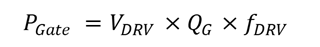The UCC53x0 comes in different variants with different drive strengths and pin configurations. The output stage of UCC5320SC, for example, has split outputs. The peak source current, IOH, is determined by VCC2 and ROH || RNMOS while the peak sink current, IOL, is determined by VCC2 and ROL, in addition to the added external turn-on and turn-off gate resistors.

We can obtain external parameters to be used as an example to calculate the peak source/sink current, please refer to section 8.11 (Electrical Characteristics) in the data sheet.

 Parameter Min Typ UNIT IOH       Peak source current 2.4 4.3 A IOL       Peak sink current 2.2 4.4 A

Table 2: UCC5320SC peak source/sink current

Additionally, we can obtain the resistances values RNMOS, ROH, and ROL from section 10.3.3 in the data sheet shown in the figure below.

 Parameter RNMOS ROH ROL UNIT UCC5320SC 4.5 12 0.65 Ohms

Table 3: UCC5320SC On-Resistance

The equations needed to calculate the expected peak source current and sink source current can be found in section 11.2.2.2 in the data sheet. The external turn-on resistance RON and the power transistor internal gate resistance RGFET(INT) reduces the peak source current as shown in the equation below. From the table above, we can use UCC5320SC parameter values as an example to calculate both the expected peak and sink source current.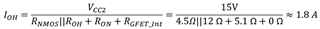• RON is the external turn-on resistance.
• RGFET_Int is the power transistor internal gate resistance, found in the power transistor data sheet. We will assume 0Ω for our example
• IOH is the peak source current which is the minimum value between 4.3 A, the gate-driver peak source current, and the calculated value based on the gate-drive loop resistance.

Similarly, the equation below is used to calculate the sink source current. The external turn-off resistance ROFF and the power transistor internal gate resistance RGFET(INT) reduces the peak sink current as shown in the equation below.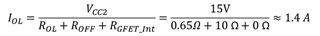• ROFF is the external turnoff resistance.
• IOL is the peak sink current which is the minimum value between 4.4 A, the gate-driver peak sink current, and the calculated value based on the gate-drive loop resistance.

From the data sheet we can obtain helpful guidelines for determining the typical capability of the gate driver performance. However, external factors such as the external gate drive resistor will impact the behavior, hence, the other sections of the datasheet should be paid attention to. To evaluate the performance of the output-high drive current across different loads of the IGBT/MOSFET, please refer to Figure 5 & 6 in the data sheet.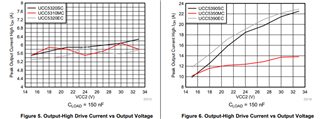• Total Gate-Driver loss dissipated

The purpose of having a gate driver is, to turn-on and turn-off the power devices efficiently. By doing so we experience switching losses as a result of the energy loss to turn the FETs on and off. Switching losses will increase with higher bias voltage, which correspondingly increases the drive current and decrease the system efficiency. Therefore, it’s critical for the gate driver to switch with minimal losses.

There are multiple factors that might affect the power loss of a gate driver; the first component is the static power loss, the second component is the switching operation loss, and the third component is the loss on the output stage.

1. Switching operating loss, PGDW, this component occurs when the capacitance of a driver charges and discharges during each switching cycle. PGDW this can be calculated using the equation below.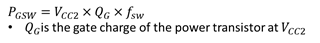2. Gate driver loss on the output stage, PGDO, in which it would be calculated using the equation below.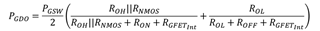The drain-source on resistance, RDS(ON) plays a role on the power dissipation of the gate driver, as mentioned earlier RDS(ON) is the parallel combination of ROH || RNMOS, from the formula above one can see the smaller RDS(ON), the smaller the gate driver loss on the output stage.

• Conclusion

This E2E explained why OUTH has a higher effective resistance than OUTL. Using a hybrid pullup structure enables our gate driver to deliver peak current during the Miller plateau region to reduce switching losses. Additionally, this report provides equations to calculate the switching power dissipation, which are essential when determining the maximum switching frequency and maximum gate capacitance the gate driver can switch. The power dissipation is determined by RDS(ON) and other factors. Since, RDS(ON) is the parallel combination of ROH || RNMOS in a hybrid pull-up structure, the power dissipation is minimized.